Function Repository Resource:

# EvenFunctionQ

Determine whether an expression is an even function of the given variable or variables

Contributed by: Wolfram|Alpha Math Team
 ResourceFunction["EvenFunctionQ"][expr,x] returns True if expr is an even function of x, and returns False otherwise. ResourceFunction["EvenFunctionQ"][expr,{x1,x2,…}] returns True if expr is an even function under the transformation {x1,x2,…}→{-x1,-x2,…}, and returns False otherwise.

## Examples

### Basic Examples (13)

Test whether a basic power function is even:

 In:=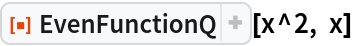Out=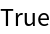Test another power function:

 In:=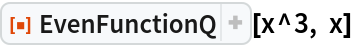Out=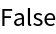Test whether a constant function is even:

 In:=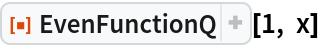Out=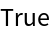Test whether the absolute value function is even:

 In:=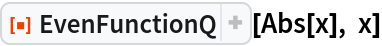Out=Test whether the sine function is even:

 In:=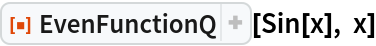Out=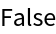Test whether the cosine function is even:

 In:=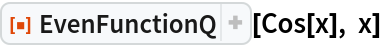Out=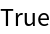Test whether a Gaussian function is even:

 In:=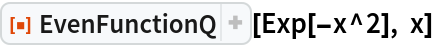Out=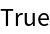Test a signed Gaussian function:

 In:=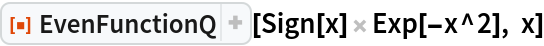Out=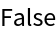Test whether a hyperbolic sine function of two variables is even:

 In:=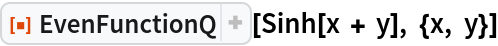Out=Test whether the hyperbolic tangent function is even:

 In:=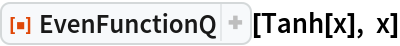Out=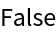Test whether the error function is even:

 In:=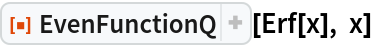Out=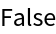Test whether a Fresnel integral is even:

 In:=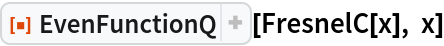Out=Test whether a shifted Fresnel integral is even:

 In:=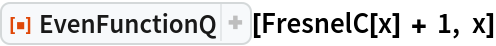Out=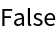### Scope (4)

For a function of multiple variables, parity is determined based on the behavior of the function under the transformation {x,y,..}{-x,-y,..}. Find the parity of a function of two variables:

 In:=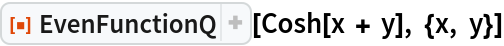Out=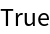In:=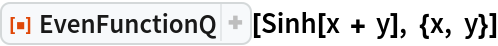Out=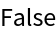Find the parity of a function of three variables:

 In:=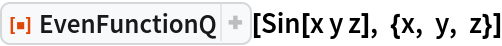Out=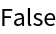Wrapping one of the variables in Abs converts this to an even function:

 In:=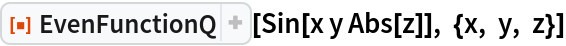Out=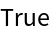Show that an implicitly-defined circle is even in both x and y:

 In:=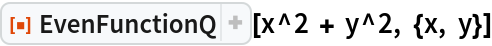Out=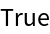## Publisher

Wolfram|Alpha Math Team

## Version History

• 4.0.0 – 23 March 2023
• 3.0.0 – 01 April 2020
• 2.0.0 – 06 September 2019
• 1.0.0 – 09 July 2019